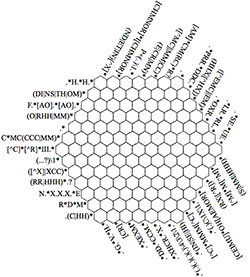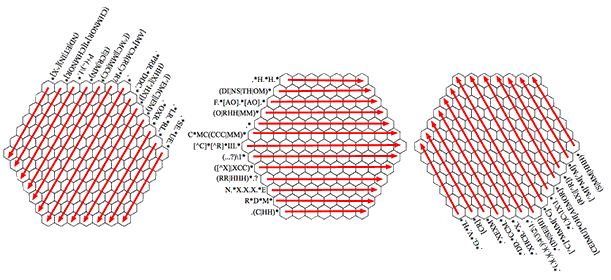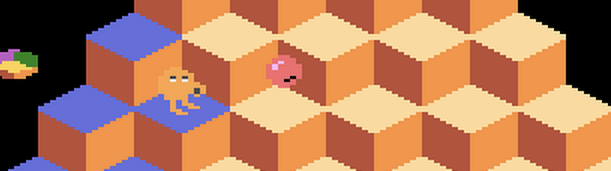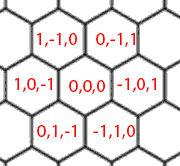# Solving a Regular Expression Crossword with Haskell, Part 2: Representation

    _   _              _    ___  _            _     _                  _
/_\ | |_ __  ___ __| |_ / _ \| |__ ___ ___| |___| |_ ___   _ _  ___| |_
/ _ \| | '  \/ _ (_-<  _| (_) | '_ (_-</ _ \ / -_)  _/ -_)_| ' \/ -_)  _|
/_/ \_\_|_|_|_\___/__/\__|\___/|_.__/__/\___/_\___|\__\___(_)_||_\___|\__|
In Glorious ASCII-VISION

Home

## 2014-02-04 Tue Solving a Regular Expression Crossword with Haskell, Part 2: Representation

In part 1 of this series I showed the Regular Expression Crossword and talked a little bit about how it works and strategies for solving it. In this part I'll show how I represented the problem space using Haskell.

Complete Haskell code for the solver is available on Github.

### A Quick RecapThe Regular Expression Crossword consists of hexagons with 3 groups of Regular Expressions, with 3 crossing on each hexagon. The objective of the game is to need to find letters for each space that match all of the expressions.

It's made a little harder by wildcards in the expressions that can match anything and by Back References that match previous parts of the expression.### Representation

Before I could get to the fun bits I needed a representation for the problem that would be relatively easy to deal with. This needed to let me:

• Store the current set of possible answers for each hexagon
• For each regular expression figure out which hexagons it needs to match against
• Easily display the current game state on screen for debugging

My first thought was to use a 2D array and refer to the hexagons by row and column. This kind of works (think of it like a square grid with every other row offset by half a square) but it has 2 problems. The first problem is that you end up with lots of positions in your array that don't match up to a square on the puzzle, but that's not too big a deal. The second problem is how to figure out which Regular Expression matches to which set of positions, have a think about it for a second and I'm sure you'll see it's a problem.

To solve this I turned to Google and quickly found this excellent article on coordinate systems for Hexagonal grids by Chris Schetter. He shows that, as expected, Q*Bert had the solution all along: use 3D coordinates!If we extend the previous 2D coordinate system to also have a third Z coordinate which is is always set to $$-(X + Y)$$. As an added bonus if we decide that the centre of the puzzle is $$(0,0,0)$$ you can find out if a point is within the puzzle by checking that none of the the coordinates has an absolute value greater than 6.

The values of each of the X, Y and Z coordinates now correspond to Regular Expressions for the three groups respectively. So if the coordinate for a hexagon was $$(-6, 2, 4)$$ you'd know that the corresponding expressions were the first, 7th and 9th from the groups (remember that our coordinates all go from -6 to 6)

You may have spotted (or read in the linked article) that we don't actually need to store one of the coordinates, it just makes everything easier to understand. So we'll only be storing the X and Y coordinates and just generating Z when it's needed.

To avoid having a spare array with lots of empty space I decided to use a Map (aka Dictionary for Python people or Object for JavaScripters) taking the (X, Y) coordinates pair as keys and Sets of letters as values

### To The Haskell-Mobile

So, finally to some Haskell! First let's define the types we'll be using to represent the puzzle (after importing the required modules).

import qualified Data.Map as Map
import qualified Data.Set as Set

-- We only store the X and Y coords, we generate Z on demand
type Coords = (Int, Int)
type Possibilities = Set.Set Char
type GameState = Map.Map Coords Possibilities


So the Coords type is a pair of Ints and is used to present the coordinates (storing just the X and Y), the Possibilities type is a Set of Characters and the GameState is a Map from Coords to Possibilities.

It's often easiest to just generate all the coordinates in the bounding square then discard any that aren't actually within the game's hexagonal play area. For this reason a way checking if a set of coordinates is within the bounds of the puzzle is needed:

validCoords :: Int -> Int -> Bool
validCoords x y = ok x && ok y && ok z
where z = -(x + y)
ok i = abs i <= 6


The starting state of the board is also needed:

allLetters = Set.fromList ['A'..'Z']

starting :: GameState
starting = Map.fromList [((x,y), allLetters) |
x <- [-6..6],
y <- [-6..6],
validCoords x y]


This uses a List Comprehension to generate all the X and Y coordinates between (-6,-6) and (6,6) then discard the ones that aren't valid. For each of the filtered coordinates a entry is made is a Map, we start off with every position containing the full set of letters (we'll narrow it down as the program runs until we get to just one letter per position).

It's always a good idea to be able to get readable debug output so here's a function to create a printable ASCII version of the board:

showGameState :: GameState -> (Int -> Int -> Int -> [Char] -> String) -> String
showGameState gamestate showCell = intercalate "\n" rows
where rows = map showRow [-6..6]
showRow x = (replicate (abs x) ' ') ++ (concatMap (showCell' x) [-6..6])
showCell' x y =
let z = -(x+y) in
case Map.lookup (x, y) gamestate of
Just c -> showCell x y z (Set.toList c) ++ " "
Nothing -> ""


How to actually display the cells is deferred to a function that is passed in, I found the most useful one would display a "." if the all 26 letters were still a possibility, a "*" if it had been narrowed down to less than that or the letter itself if answer has been found for that location. I also set it to display "!" if the set of possibilities had been reduced to none but I never saw that, the bugs manifested in different ways.

cellSolvedState :: Int -> Int -> Int -> [Char] -> String
cellSolvedState x y z chars =
case chars of
[] -> "!"
char@[_] -> char
_ -> if length chars == 26 then
"."
else
"*"


Here's the output with some sample data:

> putStrLn $showGameState currentGameState cellSolvedState * * * * E * * M * * * * * * * * M * * . * . * H H * M * * * * * * H H * * M . * * * X D C * * * * M * * * H D * C * * * * * M . . C R . R G * M * M * * * R * R * * * O . * . * . . X R N * M * M * * * E * R P O . * . * X * V * * * * * M * * * * F * M C *  What we'll mostly now want to do is to match up the regular expressions with the positions on the board that they'll need to match. regexCoordinates = xside ++ yside ++ zside where xside = [[(x,y) | y <- [-6..6], validCoords x y] | x <- [-6..6]] yside = [[(x,y) | z <- [-6..6], let x = -(y + z), validCoords x y] | y <- [-6..6]] zside = [[(x,y) | x <- [-6..6], let y = -(x + z), validCoords x y] | z <- [-6..6]]  This code generates the coordinates for each of the 3 groups of Regular Expressions separately then concatenates them all together. We can now have a single dimension array with all the Regular Expressions (first the X side, then the Y side then the Z side) and it will match up with the set of coordinates we've generated. To test it out I wrote a main function that reads in the Regular Expressions from a file, splits them up and filters out any blank lines. It then zips them up with the coordinates generated earlier and prints them all out. main = do regexFileContents <- readFile "regexs.txt" let regexs = filter (/="") (lines regexFileContents) mapM_ (putStrLn . show)$ zip regexs regexCoordinates


Which gives output like this:

(".*SE.*UE.*",[(-6,0),(-6,1),(-6,2),(-6,3),(-6,4),(-6,5),(-6,6)])
(".*LR.*RL.*",[(-5,-1),(-5,0),(-5,1),(-5,2),(-5,3),(-5,4),(-5,5),(-5,6)])
... and so on ...


Now it's just a matter of narrowing by matching the expressions against the sets of letters in the corresponding lists of coordinates. Tune in next time to hear about the custom Regular Expression engine I wrote to help do that.

Stick your email in the box below if you want an email when I add new articles to this site (including the next part). I won't use your email for anything else or let anyone else have it.

 _______________________________________
< follow me on Twitter: @almostobsolete >
---------------------------------------
\   ^__^
\  (OO)\_______
(__)\       )\/\
|| ----w|
||     ||
^^^^^^^^^^^^^^^^^^^^^^^^^^^^^^^^^


About me: I'm Tom, a freelance developer in Brighton, UK. You can hire me to write software if you want. I do Python and JavaScript mainly but I'm pretty flexible if you've got something interesting.

Date: 2014-02-04 20:26:58 GMT

Org version 7.7 with Emacs version 23

Validate XHTML 1.0